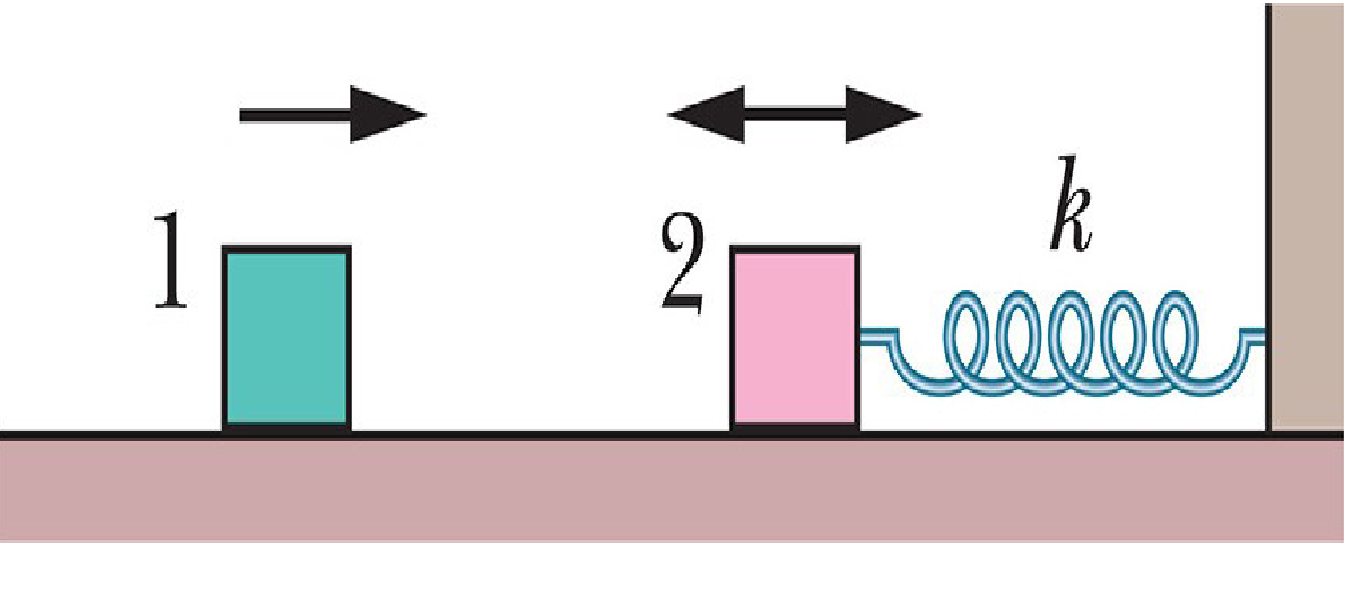# AnotherIn the Fig , block 2 of mass $2.0$ kg oscillates on the end of a spring in SHM with a period of $20$ ms. The position of the block is given by $x (t )= (1.0 cm)\ cos (\omega t + \pi/2)$ . Block $1$ of mass$4.0$kg slides toward block $2$ with a velocity of magnitude $6.0$m/s, directed along the spring’s length.The two blocks undergo a completely inelastic collision at time $t = 5.0$ms. (the duration of the collision is much less than the period of motion.) What is the amplitude of the SHM after the collision?

×